Processing ......FreeComputerBooks.com Links to Free Computer, Mathematics, Technical Books all over the World

Applied Mathematics
Related Book Categories:
 Bayesian Thinking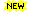Control Theory and Systems Algebra, Abstract and Linear Algebra, etc. Fourier Transforms and Wavelet Geometry and Topology Numerical Analysis and Scientific Computing Operations Research and Optimization Probability and Stochastic Discrete and Finite Mathematics Mathematical MusicMathematical and Computational Software Game TheoryDifferential Equations Calculus and Mathematical Analysis Digital Signal Processing (DSP) Graph Theory Statistics Physics Number Theory Cryptography, Cryptology, and Cryptanalysis Financial Mathematics and Engineering Computational and Algorithmic Mathematics
•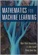Mathematics for Machine Learning (Marc P. Deisenroth, et al.)

This self contained textbook bridges the gap between mathematical and machine learning texts, introducing the mathematical concepts with a minimum of prerequisites. It provides a beautiful exposition of the mathematics underpinning modern machine learning.

•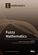Fuzzy Mathematics (Etienne E Kerre, et al)

This book provides a timely overview of topics in fuzzy mathematics. It contains not only theoretical results, but a wide range of applications in different areas, as well as an introduction into the fascinating topic of Fuzzy Sets and Fuzzy Logic.

•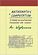Mathematics and Computation (Avi Wigderson)

This book provides a broad, conceptual overview of computational complexity theory - the mathematical study of efficient computation. With important practical applications to computer science and industry, computational complexity theory, etc.

•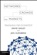Networks, Crowds, and Markets: a Highly Connected World

It looks at economics, sociology, computing and information science, and applied mathematics to understand networks and behavior, and addresses fundamental questions about how the social, economic, and technological worlds are connected.

•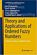Theory and Applications of Ordered Fuzzy Numbers

This book offers comprehensive coverage on Ordered Fuzzy Numbers, providing readers with both the basic information and the necessary expertise to use them in a variety of real-world applications.

•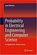Probability in Electrical Engineering and Computer Science

This textbook motivates and illustrates the techniques of applied probability by applications in electrical engineering and computer science (EECS). Python labs enable the readers to experiment and consolidate their understanding.

•Foundations of Fuzzy Logic and Semantic Web Languages

This book provides a rigorous and succinct account of the mathematical methods and tools used for representing and reasoning with fuzzy information within Semantic Web languages. The book focuses on the three main streams of Semantic Web languages.

•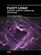Fuzzy Logic - Controls, Concepts, Theories and Applications

This book introduces new concepts and theories of Fuzzy Logic Control for the application and development of robotics and intelligent machines - how it will play a significant role in the development of intelligent robotic, machine, and mechatronics systems.

•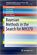Bayesian Methods in the Search for MH370 (Samuel Davey, et al.)

This book demonstrates how nonlinear/non-Gaussian Bayesian time series estimation methods were used to produce a probability distribution of potential MH370 flight paths, which was used to define the search zone in the southern Indian Ocean.

•Mathematical Foundations of Quantum Theory

This is a collection of lecture notes, all shared a common interest in answering quantum issues. The goal is to give a mathematically clear and self-containing explanation of the main concepts of the modern language of quantum theory.

•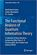The Functional Analysis of Quantum Information Theory

This book provides readers with a concise introduction to current studies on operator-algebras and their generalizations, operator spaces and operator systems, with a special focus on their application in Quantum Information Science.

•A Basic Course in the Theory of Interest and Derivatives Markets

This book is designed for an introductory course in the theory of interest and annuity. Each section contains the embedded examples with answer keys. It is suitable for a junior level course in the mathematics of finance.

•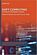Soft Computing: Techniques in Engineering Sciences

Soft Computing is used where a complex problem is not adequately specified for the use of conventional math and computer techniques. This book elaborates on the most recent applications of Soft Computing in various fields of engineering.

•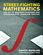Street-Fighting Math: Guessing & Opportunistic Problem Solving

This engaging book is an antidote to the rigor mortis brought on by too much mathematical rigor, teaching us how to guess answers without needing a proof or an exact calculation.

•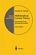Mathematical Control Theory (Eduardo D. Sontag)

This book covers what constitutes the common core of control theory and is unique in its emphasis on foundational aspects. While covering a wide range of topics written in a standard theorem/proof style, it also develops the necessary techniques from scratch.

•Feedback Control Theory (John C. Doyle, et al)

An excellent introduction to feedback control system design, this book offers a theoretical approach that captures the essential issues and can be applied to a wide range of practical problems.

•Feedback Systems: An Introduction for Scientists and Engineers

This book provides an introduction to the mathematics needed to model, analyze, and design feedback systems. It uses techniques from physics, computer science, and operations research to introduce control-oriented modeling.

•Variational Principles in Classical Mechanics (Douglas Cline)

This book introduces variational principles and their application to classical mechanics. The relative merits of the intuitive Newtonian vectorial formulation, and the more powerful variational formulations are compared.

•Statistical Methodologies and Their Application to Real Problems

This book provides a cross-disciplinary forum for exploring the variety of new data analysis techniques emerging from different fields, focusing on recent advances in data analysis techniques in many different research fields.

•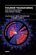Fourier Transforms - High-tech Application and Current Trends

The main purpose of this book is to provide a modern review about recent advances in Fourier transforms as the most powerful analytical tool for high-tech application in electrical, electronic, and computer engineering, as well as other applications.

•Category Theory for the Sciences (David I. Spivak)

Using databases as an entry to category theory, this book explains category theory by examples, and shows that category theory can be useful outside of mathematics as a rigorous, flexible, and coherent modeling language throughout the sciences.

•An Invitation to Applied Category Theory: Seven Sketches

Category theory is now a powerful tool in science, informatics, and industry. This book offers a self-contained tour of applied category theory. Each chapter follows a single thread motivated by a real-world application and discussed with category-theoretic tools.

•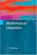Mathematical Linguistics (Andras Kornai)

This book introduces the mathematical foundations of linguistics to computer scientists, engineers, and mathematicians interested in natural language processing (NLP). It presents linguistics as a cumulative body of knowledge from the ground up.

•Math Alive (Ingrid Daubechies, et at)

Mathematics has profoundly changed our world, from banking to listening to music. This course is designed for those who haven't had college mathematics but would like to understand some of the mathematical concepts behind important modern applications.

•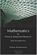Mathematics for Classical Information Retrieval: Roots and Apps

This book is about Information Retrieval (IR), particularly Classical Information Retrieval (CIR). The primary goal of book is to create a context for understanding the principles of CIR by discussing its mathematical bases.

•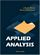Applied Analysis (John K. Hunter, et al)

This book provides an introduction to those parts of analysis that are most useful in applications for graduate students. The material is selected for use in applied problems, and is presented clearly and simply but without sacrificing mathematical rigor.

•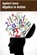Applied Linear Algebra in Action (Vasilios N. Katsikis)

This textbook contains a collection of six high-quality chapters. The techniques are illustrated by a wide sample of applications. It is devoted to Linear Mathematics by presenting problems in Applied Linear Algebra of general or special interest.

•Applied Linear Algebra: Vectors, Matrices, and Least Squares

Combines straightforward explanations with a wealth of practical examples to offer an innovative approach to teaching linear algebra. Requiring no prior knowledge of the subject, it covers the aspects of linear algebra - vectors, matrices, and least squares

•Linear Algebra: A Course for Physicists and Engineers (Arak Mathai)

This textbook on linear algebra is written to be easy to digest by non-mathematicians. It introduces the concepts of vector spaces and mappings between them without too much theorems and proofs. Various applications of the formal theory are discussed as well.

•Analysis and Linear Algebra for Finance (Patrick Roger)

This book presents the elements of analysis and linear algebra used in financial models and in microeconomics. Functions of one and several variables and matrices are developed as well as vector spaces, linear mappings and optimization methods, etc.

•Applied and Computational Linear Algebra: A First Course

This book is intended as a text for a graduate course that focuses on applications of linear algebra and on the algorithms used to solve the problems that arise in those applications.

•Applied Finite Mathematics (Rupinder Sekhon)

This book covers topics including linear equations, matrices, linear programming (geometrical approach and simplex method), the mathematics of finance, sets and counting, probability, Markov chains, and game theory.

•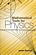Mathematical Tools for Physics (James Nearing)

This book helps physics students learn to take an informed and intuitive approach to solving problems. It assists undergraduates in developing their skills and provides them with grounding in important mathematical methods.

•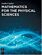Mathematics for the Physical Sciences (Leslie Copley)

This book provides a comprehensive introduction to the areas of mathematical physics. It combines all the essential math concepts into clearly written reference and illustrates the mathematics with numerous physical examples drawn from contemporary research.

•Mathematics for the Physical Sciences (Herbert S. Wilf)

This book provides a text for a first-year graduate level course in mathematical methods. Advanced undergraduates and graduate students in the natural sciences will receive a solid foundation in several fields of mathematics with this text.

•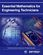Essential Mathematics for Engineers (W. J. R. H. Pooler)

This book explains mathematical jargon and develops the formulae used by engineers from first principles. The first chapter is a summary so the reader can quickly see where further study is needed. The book is in two parts, pure and applied.

•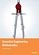Essential Engineering Mathematics (Michael Batty)

The aim is more to highlight and explain some areas commonly found difficult, such as calculus, and to ease the transition from school level to university level mathematics, where sometimes the subject matter is similar, but the emphasis is usually different.

•Mathematics for Computer Science (Eric Lehman, et al)

This book covers elementary discrete mathematics for computer science and engineering. It emphasizes mathematical definitions and proofs as well as applicable methods. explores the topics of basic combinatorics, number and graph theory, logic and proof techniques.

•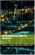Mathematics for Computer Scientists (Gareth J. Janacek, et al)

The aim of this book is to present some the basic mathematics that is needed by computer scientists. The reader is not expected to be a mathematician and we hope will find what follows useful.

•Shape Interrogation for Computer Aided Design and Manufacturing

This book provides the mathematical fundamentals as well as algorithms for various shape interrogation methods including nonlinear polynomial solvers, intersection problems, differential geometry of intersection curves, distance functions etc.

•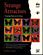Strange Attractors: Creating Patterns in Chaos (Julien C. Sprott)

This book describes a simple method for generating an endless succession of beautiful fractal patterns by iterating simple maps and ordinary differential equations with coefficients chosen automatically by the computer.

•Mathematics in the Age of the Turing Machine (Thomas C. Hales)

Computers have rapidly become so pervasive in mathematics that future generations may look back to this day as a golden dawn. The article gives a survey of mathematical proofs that rely on computer calculations and formal proofs.

•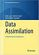Data Assimilation: A Mathematical Introduction (Kody Law, et al)

This book provides a systematic treatment of the mathematical underpinnings of work in data assimilation, covering both theoretical and computational approaches. Specifically the authors develop a unified mathematical framework of Bayesian formulation.

•Mathematics for Algorithm and Systems Analysis (E. A. Bender)

This up-to-date textbook assists undergraduates in mastering the ideas and mathematical language to address problems that arise in the field's many applications.

•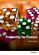Probability for Finance (Patrick Roger)

This book provides technical support for students in finance. It reviews the main probabilistic tools used in financial models in a pedagogical way, starting from simple concepts like random variables and tribes and going to more sophisticated ones like conditional expectations and limit theorems.

•Stochastic Processes for Finance (Patrick Roger)

It describes the most important stochastic processes used in finance in a pedagogical way, especially Markov chains, Brownian motion and martingales. It also shows how mathematical tools like filtrations, Ito's lemma or Girsanov theorem should be understood in the framework of financial models.

•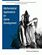Mathematical Applications for Game Development (R. Baldwin)

This book presents applications of mathematics and science in game and simulation programming. Includes the utilization of matrix and vector operations, kinematics, and Newtonian principles in games and simulations. Also covers code optimization.

•Vector Math for 3D Computer Graphics, 4th Revision (Bradley Kjell )

This is a tutorial on vector algebra and matrix algebra from the viewpoint of computer graphics. It covers most vector and matrix topics needed to read college-level computer graphics text books.

•3D Math Primer for Graphics and Game Development (F. Dunn)

Working C++ classes for mathematical and geometric entities and several different matrix classes illustrate how to put the techniques into practice, and exercises at the end of each chapter help reinforce the 3D programming concepts.

•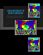Linear Mathematics In Infinite Dimensions (Ulrich H. Gerlach)

Focus on the mathematical framework that underlies linear systems arising in physics, engineering and applied mathematics - from the theory of linear transformation on finite dimensional vector space to the infinite dimensional vector spaces.

•Numerical Simulations of Physical and Engineering Processes

The book handles the numerical simulations of physical and engineering systems. It can be treated as a bridge linking various numerical approaches of two closely inter-related branches of science, i.e. physics and engineering.

•Games, Fixed Points and Mathematical Economics (C. Ewald)

This book gives the reader access to the mathematical techniques involved and goes on to apply fixed point theorems to proving the existence of equilibria for economics and for co-operative and noncooperative games.

•Call Center Mathematics: Understanding and Improving

This book gives an overview of the role and potential of mathematical optimization in call centers. It deals extensively with all aspects of workforce management, but also with topics such as call routing and the scheduling of multiple channels.

•Modern Signal Processing (Daniel N. Rockmore, et al)

Signal processing is ubiquitous in modern technology. Its mathematical basis and applications are the subject of this book. Emphasis is on challenges, new techniques adapted to new technologies, and certainrecent advances in algorithms and theory.

•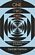One Two Three ... Infinity: Facts and Speculations of Science

Whatever your level of scientific expertise, chances are you'll derive a great deal of pleasure, stimulation, and information from this unusual and imaginative book. It belongs in the library of anyone curious about the wonders of the scientific universe.

•Semantics - Advances in Theories and Mathematical Models

This book is a nice blend of number of great ideas, theories, mathematical models, and practical systems in the domain of Semantics, which highlights the advances in theories and mathematical models in the domain of Semantics.

•Mathematics and Music (David Wright)

An investigation of the interrelationships between mathematics and music, reviewing the needed background concepts in each subject as they are encountered. It explores the common foundations of the two subjects, which are developed side by side.

•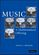Music: A Mathematical Offering (David J. Benson)

Starting with the structure of the human ear and its relationship with Fourier Analysis, this book provides a wealth of information to understand, at varying levels of technicality, the interplay between two ancient disciplines.

•How Music and Mathematics Relate (David Kung)

Understanding the connections between music and mathematics helps you appreciate both, even if you have no special ability in either field - from knowing the math behind tuning an instrument to understanding the features that define your favorite pieces.

•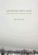Modeling with Data: Tools and Techniques for Scientific Computing

Modeling with Data fully explains how to execute computationally intensive analyses on very large data sets, showing readers how to determine the best methods for solving a variety of different problems, etc. with powerful statistical and mathematical tools.

Book Categories
 :All CategoriesTop BooksRecent BooksMiscellaneous BooksComputer LanguagesComputer ScienceData Science/DatabasesElectrical EngineeringJava and Java EE (J2EE)Linux and UnixMathematicsMicrosoft and .NETMobile ComputingNetworking and CommunicationsSoftware EngineeringSpecial TopicsWeb Programming
Other Categories